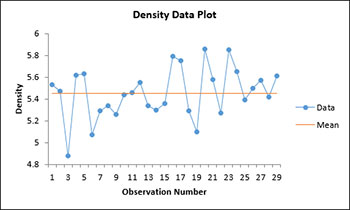# One Sample Variance Test HelpThis test is performed on the variance of one sample. The test gives you a confidence interval for the standard deviation and the variance. It has the option to test the hypothesis that the standard deviation and variance is equal to, less than, or greater than a specified standard deviation and variance. You also have the option for a two-sided, lower one-sided or an upper one-sided test.

The data can be in a range in a worksheet or the user can enter an average, standard deviation, and sample size for the test. The example on this page demonstrates how to do this test using data in a range in the worksheet.

1. Enter the data into a worksheet as shown below. The data can be one column, one row, or in multiple rows/columns. The data can be downloaded at this link.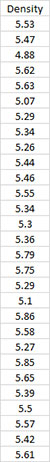2. Select the data including the heading.

3. Select “Sample Tests” from the “Statistical Tools” panel in the SPC for Excel ribbon.

4. Select the “One Sample Variance Test” option from the “Sample Tests” form. Select OK and the input form below is displayed.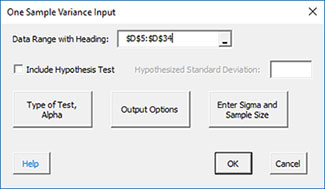• Data Range with Heading: this is the worksheet range containing the data and the heading; the default is the selected worksheet range.
• Include Hypothesis Test: this option will include a hypothesis test in addition to the confidence interval. If you select this option, you must include a hypothesized standard deviation.
• Type of Test, Alpha: the input form below is displayed if this option is selected.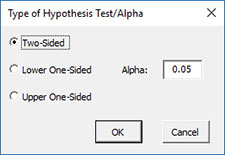• Select the Type of Test: two-sided; lower one-sided, or upper one-sided, the default is two-sided.
• Alpha: this is the confidence level; 1-alpha is the confidence interval, default is 0.05 or 95% confidence interval.
• Output Options: the input form below is displayed if this option is selected.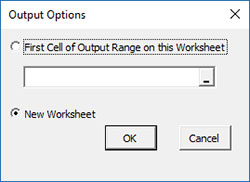.

• First Cell of Output Range on this Worksheet: Select this option if you want the output on an existing worksheet; then select the first cell of the range where you want the output placed; a check is made to ensure that no existing data are overwritten in the worksheet.
• New Worksheet: Select this option if you want the results on a new worksheet.
• Enter Sigma and Size: the input form below is displayed if this option is selected.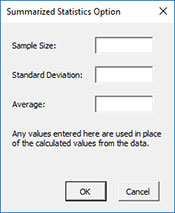• You many enter the sample size and standard deviation any value that is entered will be used in place of the values calculated from the data.
• Select OK to generate the results.
• Select Cancel to end the program.

One Sample Variance Test Output

The output from the example data (using a hypothesize standard deviation = 0.23) is shown below. An explanation of terms is given below the output.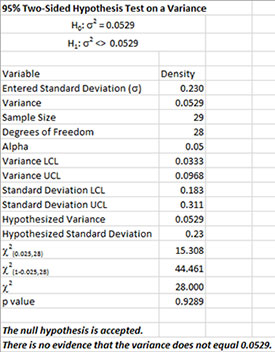The output tells you the conclusion from the test. The null hypothesis (H0) and the alternate hypothesis (H1) are printed below the title.

• Variable: Name of variable
• Standard Deviation: Calculated standard deviation
• Variance: Calculated variance
• Sample Size: Number of data points
• Degrees of Freedom: Degrees of freedom = n – 1
• Alpha: Value of alpha entered by user
• Variance LCL: (1-alpha)% lower confidence interval for the variance
• Variance UCL: (1-alpha)% upper confidence interval for the variance
• Standard Deviation LCL: (1-alpha)% lower confidence interval for the standard deviation
• Standard Deviation UCL: (1-alpha)% upper confidence interval for the standard deviation
• Hypothesized Variance: Square of specified standard deviation entered by user
• Hypothesized Standard Deviation: Specified standard deviation by user
• Chi Square(0.025,28): Lower Chi Square value based on alpha and the degrees of freedom
• Chi Square (1-0.025,28): Upper Chi Square value based on alpha and the degrees of freedom
• Chi Square: Calculated Chi Square value
• p value: Calculated p value (in red if p value ? alpha)

The program also creates a chart showing the confidence interval, the variance and hypothesized variance (if included).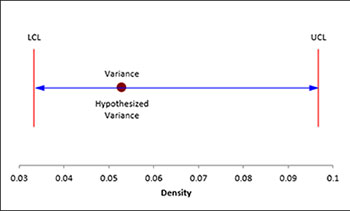There is also a chart of the data to look for possible outliers. If the program detects possible outliers, they will be in red and a message will be printed on the worksheet.